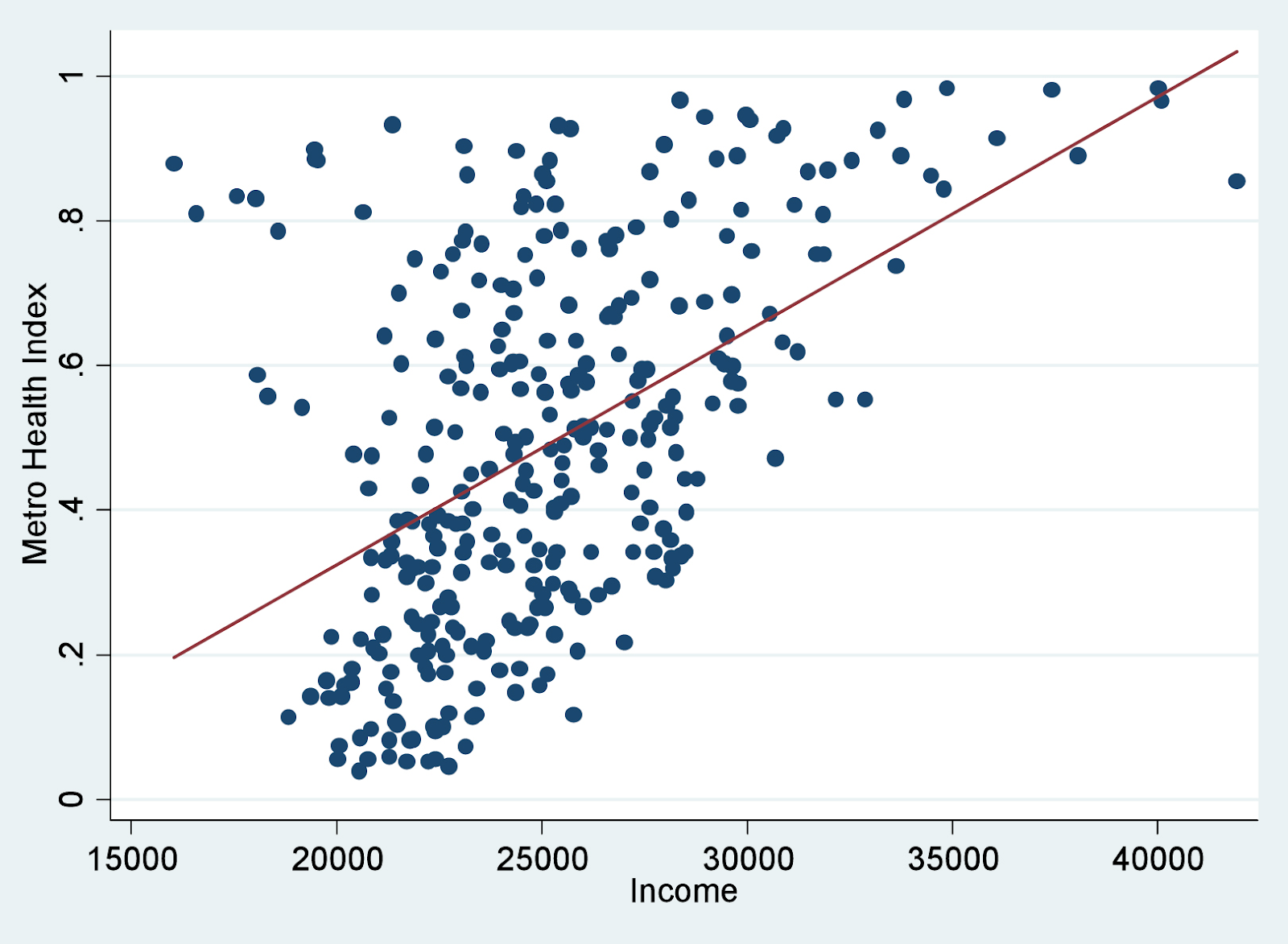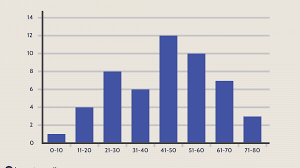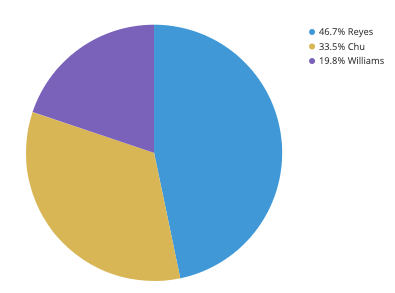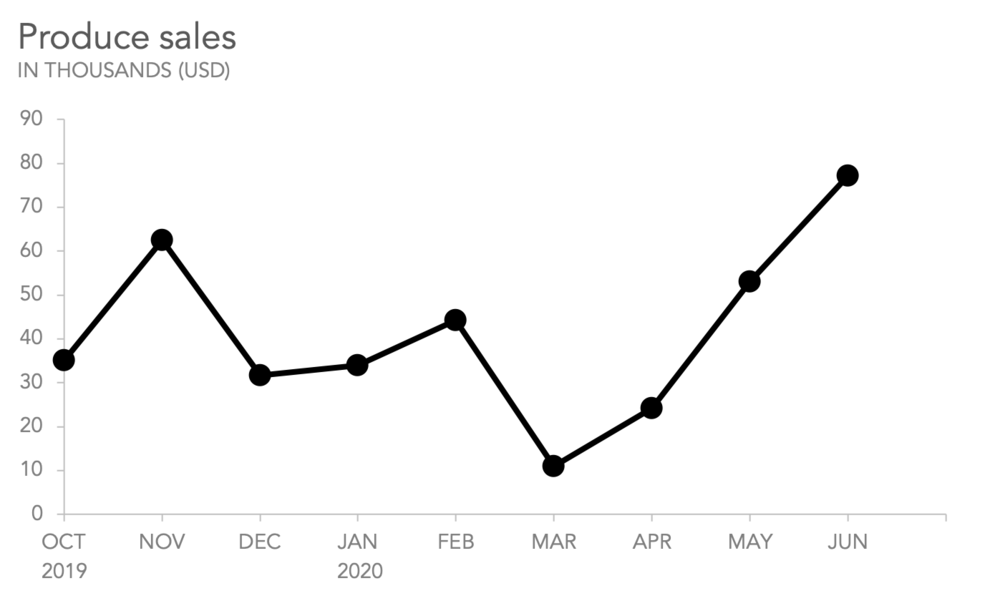Introduction to Data Visualisation

Data visualisation is one of the most important skills of data scientists. Data visualization makes it easier to understand the data and draw conclusions from data. Data visualisation also helps to understand its distribution and various statistical measures related to it.

There are various tools in python for data visualisation, namely

1. Matplotlib
2. Seaborn
3. Plotly
4. ggplot

There are other libraries too, but the above ones are most commonly used.

NUMERICAL DATA

Scatter Plot

A Scatter plot is used to determine the correlation between two variables to determine whether the variables depend on each other or not.

It is generally used for visualising data for classification.Histogram

A histogram is made up of bars, generally used to represent the frequency distribution in successive class intervals.

Histogram involves distributing the x-axis into bins and plotting a bar representing the frequency using a bar. Choosing the number of bins may significantly affect the visualisation created.

Remember, histograms are generally used for continuous data.

For example, it can be used to represent frequency distribution according to age groups in a city.Pie Charts

Pie charts are generally used to represent proportions of a quantity. A slice represents the proportion of that quantity out of the whole pie. Pie charts are a little less informative and hence are seldom used.

For example, it can be used to represent the proportion of boys and girls in a classroom.Line Charts

Line charts are generally used to represent trends. It is used to represent a change in a particular quantity with a change in the other quantity. It can be used to plot time-series graphs, i.e., change in particular quantity with the time change.

It is ubiquitous to see line graphs represent stock prices over time.Box Plots

Box plots are very informative and provide a statistical overview of the data. It represents the median, quartiles, maximum, and minimum on the plot itself.

It is generally used when a statistical overview of the data is required.

TEXTUAL DATA

Word Clouds

Word clouds provide a good way of representing textual data for visualization. According to their frequencies, word clouds sort the words according to their frequencies inside the corpus and print those words beautifully in sizes.

It helps to understand what words are used most often in the corpus. However, it is best to remove most common words like the, is, etc, before making a word cloud.

Naturally, histograms and other methods can also be used after calculating the frequencies, but here we consider numerical data.•  January, 13, 2021